## MATHS :: Lecture 03 :: Bionomial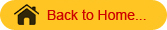BINOMIAL THEOREM
A Binomial is an algebraic expression of two terms which are connected by the operation  ‘+’ (or) ‘-‘
For examplex+siny, 3x2+2x, cosx+sin x etc… are binomials.
Binomial Theorem for positive integer:
If n is a positive integer then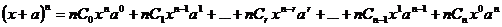----(1)

Some Expansions
a) If we put a = -a in the place of   a in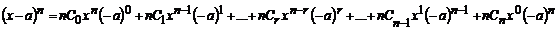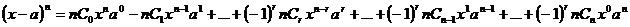b) Put  x =1 and a = x in (1)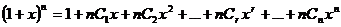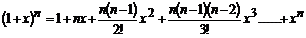----------(2)
c) Put  x = 1 and  a = -x in (1)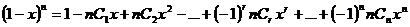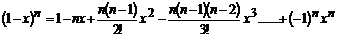-----------(3)
(d) Replacing n by – n in equation (2)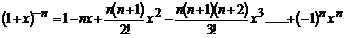---------(4)
e) Replacing n by – n in equation (3)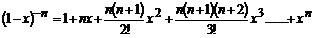-----------(5)
Special Cases
1.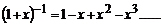2.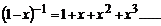3.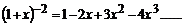4.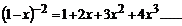Note:
1. There are n+1 terms in the expansion of (x+a)n.
2. In the expansion the general term is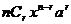. Since this is the (r+1)th term, it is denoted by Tr+1 i.e.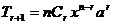.
3.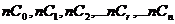are called binomial coefficients.
4. From the relation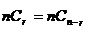, we see that the coefficients of terms equidistant from the beginning and the end are equal.
Note:  The  number of terms in the expansion of (x+a)n depends upon the index n. the index is  either even (or) odd. Then the middle term is
Case(i): n is even
The number of terms in the expansion is (n+1), which is odd.
Therefore, there is only one middle term and is given by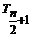Case(ii) : n is odd
The number of terms in the expansion is (n+1), which is even.
Therefore, there are two middle terms and they are given by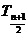and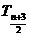Examples
1. Expand   (i)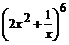2. Find 117.
Solution:
117=  (1+10)7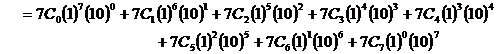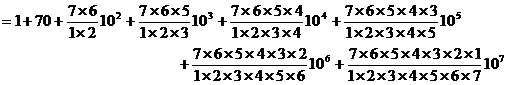= 1+ 70 + 2100 +35000 + 350000 + 2100000 + 7000000 + 10000000
= 19487171

2. Find the coefficient of x5 in the expansion of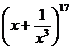Solution
In the expansion of, the general term is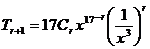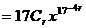Let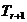be the term containing x5
then, 17-4r = 5 Þ r = 3=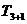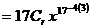= 680 x5
\coefficient of x5 = 680.
3. Find the constant term in the expansion of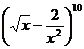Solution
In the expansion of, the general term is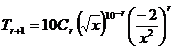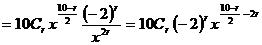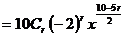Letbe the Constant term then,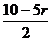= 0Þ r = 2
\ The constant term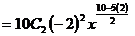=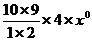= 180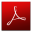Download this lecture as PDF here